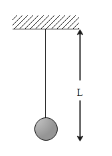As the temperature is increased, the time period of a pendulumA. increases as its effective length increases even though its centre of mass still remains at the centre of the bob. B. decreases as its effective length increases even though its centre of mass still remains at the centre of the bob. C. increases as its effective length increases due to shifting of centre of mass below the centre of the bob. D. decreases as its effective length remains the same but the centre of mass shifts above the centre of the bob.Verified
145.8k+ views
Hint: To solve this question, we first need to understand the basics of a pendulum, its bob and the material it is made of. Depending on this information, we can determine if its volume increases with temperature and what effects this will have on its time period.

Let us first define the meaning of simple pendulum. A simple pendulum is defined as an ideal pendulum which consists of a point mass suspended by a weightless inextensible perfectly flexible thread and then the freedom to oscillate without friction. This is how it can be distinguished from a physical pendulum.

The time period of a simple pendulum is defined as the time for one complete cycle, a left swing and a right swing, which is called a period. The time period of a pendulum, depends on the length of the pendulum and also to a slight degree on the amplitude, the width of the pendulum’s swing.
The time period of a simple pendulum is defined by the given formula:

$T=2\pi \sqrt{\dfrac{L}{g}}$ ………. (1)

In the above formula, L represents the effective length of the pendulum and g is the acceleration due to gravity.
We know that with an increase in temperature, the effective length or L of the simple pendulum increases. This increment happens even when the centre of mass still remains at the centre of the bob.
From the equation (1) we can say that $T\alpha \sqrt{L}$
Therefore, the T increases as the temperature increases.

So, the correct answer is “Option A”.

Note:
We must take care of the amplitude in a pendulum which changes with change in properties of the environment or weight of the bob. Length of the string doesn’t play any role in the change of amplitude as it has been assumed as light and inextensible.